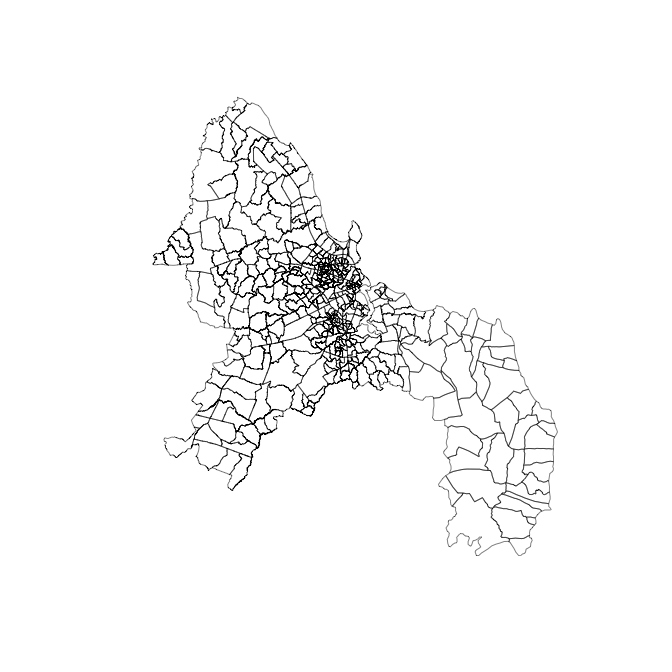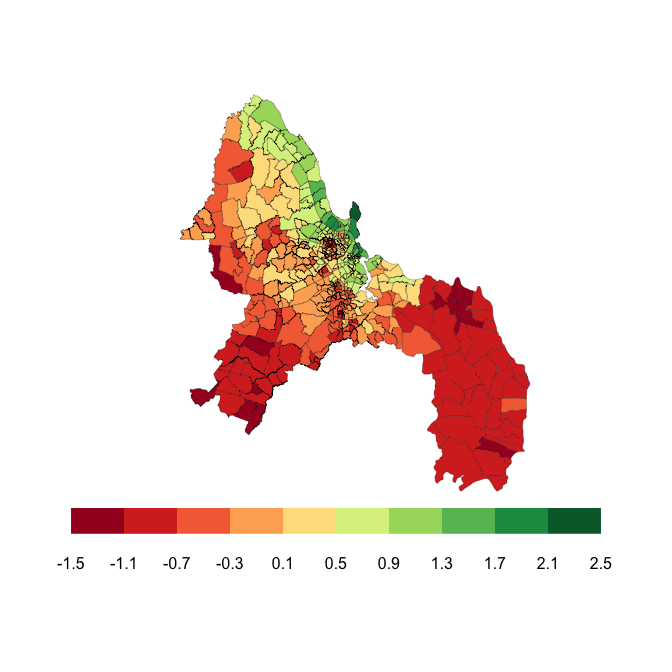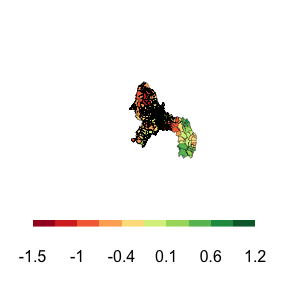# BSBT for Dar es Salaam

BSBT (Bayesian Spatial Bradley–Terry) is a package which fits a Bradley–Terry model with a spatial component for comparative judgement data sets. This can be used to estimate the quality of areas used in the data set.

In this vignette, we’ll use the BSBT package to estimate the levels of deprivation in different parts of Dar es Salaam, Tanzania.

The package includes shapefiles for the 452 subwards in Dar es Salaam. We load them by calling

data("dar.shapefiles")
library(sf)
#> Linking to GEOS 3.8.1, GDAL 3.1.4, PROJ 6.3.1
plot(dar.shapefiles$geometry, lwd = 0.5)The library sf allows us to plot the shapefiles. Also included in the package is the adjacency matrix (although it is possible to recreate this in a number of ways, eg the surveillance package). We load it by calling data("dar.adj.matrix") The $$(i,j)^{th}$$ element of the adjacency matrix is 1 if subwards $$i$$ and $$j$$ are neighbors and 0 otherwise. We manually include two extra pairs of neighbors to allow for connections across the Kurasini creek, which flows through the city. The adjacency matrix allows us to view the city as a network where the subwards are nodes and edges are placed between neighboring subwards. The advantage to this is that it makes the density of subwards uniform across the city. The center of Dar es Salaam is densely packed with small subwards, whereas on the outskirts the subwards are much larger. This range of subward sizes and densities means using the Euclidean metric is not suitable, and the network version of the city resolves these issues. The comparative judgement data set is also included in the package. It can be loaded using the command data("dar.comparisons") The data set consists of 75,078 comparisons, where judges were shown a pair of subwards and asked to choose which of the pair was more deprived. Some of the comparisons are shown below. In the first comparison subward 359 was judged to be more derived than subward 196. head(dar.comparisons) #> poorest richest gender #> 1 359 196 male #> 2 222 87 male #> 3 330 87 male #> 4 34 30 male #> 5 222 395 male #> 6 287 397 male The BSBT package requires the data in a matrix, where the $$(i, j)^{th}$$ element contains the number of times area $$i$$ was judged to be more deprived than area $$j$$. We construct the matrix using the following code win.matrix <- BSBT::comparisons_to_matrix(452, dar.comparisons) ## Constructing the Prior Distribution Covariance Matrix The multivariate normal prior distribution covariance matrix is an important part of the method. This allows us to specify how the deprivation parameters in difference parts of the city are correlated. We use the function constrained_adjacency_covariance_function to construct this matrix, which is called by k <- constrained_adjacency_covariance_function(dar.adj.matrix, type = "matrix", hyperparameters = c(1), linear.combination = rep(1, 452), linear.constraint = 0) In this example, we construct the covariance matrix using the matrix exponential of the adjacency matrix. The assigns high covariance to subwards which are highly connected (having lots of short paths connecting them) and low covariance to subwards which are only connected through long or convoluted paths. We set the variance hyperparameter to 1, as we are going to learn this value in the MCMC algorithm. As the BSBT model is a comparative judgement model, there is a identifiability issue. To resolve this we can fix a linear combination of the deprivation levels. This takes the form $$\boldsymbol{A\lambda} = a$$, where $$\boldsymbol{A}$$ is a vector containing the coefficient of the linear combination, $$\boldsymbol{\lambda}$$ is the vector of deprivation levels and $$a$$ is the value of the constraint. In our example above, we set $$\boldsymbol{A} = \boldsymbol{1}$$ and $$a = 0$$, which is equivalent to the sum of the levels being 0. ## Fitting the Model We fit the model using an MCMC algorithm. This is called by the following function set.seed(123) #this seed reproduces the results in the paper mcmc.output <- run_mcmc(n.iter = 1500000, delta = 0.01, covariance.matrix = k, win.matrix, f.initial = rep(0, 452), alpha = TRUE) This takes around 2.5 hours to run, so we do not call it in this vignette. However, we include the results for $$f$$ for this seed (123). A burn-in period of 500,000 iterations was used. The results can be loaded by data("mean.deprivation") We now produce a map of Dar es Salaam, colouring each subward by its posterior mean deprivation level. We create 10 equal sized bins in which to place the subwards and colour the bins appropriately.  #Create Colour Scale library(RColorBrewer) red.green.colours <- brewer.pal(10, "RdYlGn") bin.size <- (2.5-(-1.5))/10 bins <- bin.size*(1:10) - 1.5 #Bin Subwards by colour dar.colours <- numeric(452) for(j in 1:452){ dar.colours[j] <- min(which(bins >= mean.deprivation[j])) } To plot the map, we call: oldpar <- par() #save current graphical parameter par(fig=c(0,1,0.1,1)) plot(dar.shapefiles$geometry, col = red.green.colours[dar.colours], lwd = 0.25)
par(fig=c(0.1,0.9,0.2,0.25), mar = rep(0.2, 4), new = TRUE)
image(1:10, 1, as.matrix(1:10), col = brewer.pal(10, "RdYlGn"),
xlab = "", ylab = "", xaxt = "n", yaxt = "n",
bty = "n")
axis(1, at = seq(0.5, 10.5, 1), labels = round(c(-1.5, bins), 2.5), lty = 0)par(fig = oldpar$fig) #reset graphical parameters ## Differing Opinions of Men and Women As we have the gender of each judge, we can investigate if the male and female judges had differing opinions. This is important to investigate in developing countries, as women often face risks, such as FGM or forced marriage, and we identify areas where women face these risks. We begin by splitting the comparisons based on the judges reported gender and constructing separate win/loss matrices for men and women. male.comparisons <- dar.comparisons[dar.comparisons$gender == "male", ]
female.comparisons <- dar.comparisons[dar.comparisons$gender == "female", ] male.win.matrix <- matrix(0, 452, 452) for(j in 1:dim(male.comparisons)) male.win.matrix[male.comparisons[j, 1], male.comparisons[j, 2]] <- male.win.matrix[male.comparisons[j, 1], male.comparisons[j, 2]] + 1 female.win.matrix <- matrix(0, 452, 452) for(j in 1:dim(female.comparisons)) female.win.matrix[female.comparisons[j, 1], female.comparisons[j, 2]] <- female.win.matrix[female.comparisons[j, 1], female.comparisons[j, 2]] + 1 We then construct the prior distribution covariance matrices, one for the grand mean and one for the difference between the genders. We assume each matrix has the same structure, but allow for different variance parameters. k <- constrained_adjacency_covariance_function(dar.adj.matrix, type = "sqexp", hyperparameters = c(1, 0.5), linear.combination = rep(1, 452), linear.constraint = 0) We can then run the MCMC algorithm to estimate the model parameters. We include the code here, but do not run it in the vignette due to time constraints. mcmc.output <- run_gender_mcmc (2000000, 0.15, rep(0, 452), covariance.matrix, male.win.matrix, female.win.matrix, rep(0, 452), rep(0, 452)) The grand mean is given by f and the difference between the genders by g. To plot the difference in perceptions between the men and the women, we call data("male.mean.deprivation") data("female.mean.deprivation") g <- female.mean.deprivation - male.mean.deprivation bin.size <- (max(2.01*g) - min(2*g))/10 bins <- bin.size*(1:10) + min(2*g) diff.colours <- numeric(452) for(j in 1:452) diff.colours[j] <- min(which(bins >= 2*g[j])) oldpar <- par() #save current graphical parameter par(fig=c(0,1,0.1,1)) plot(dar.shapefiles$geometry, col = red.green.colours[diff.colours], lwd = 0.25)
par(fig=c(0.1,0.9,0.2,0.25), mar = rep(0.2, 4), new = TRUE)
image(1:10, 1, as.matrix(1:10), col = brewer.pal(10, "RdYlGn"),
xlab = "", ylab = "", xaxt = "n", yaxt = "n",
bty = "n")
axis(1, at = seq(0.5, 10.5, 1), labels = round(c(min(2*g), bins), 1), lty = 0)par(fig = oldpar\$fig) #reset graphical parameters

The red areas are percieved as more deprived by the women and the red areas are viewed as more deprived by the men.